# JavaScript 游戏开发：手把手实现碰撞物理引擎

### 目录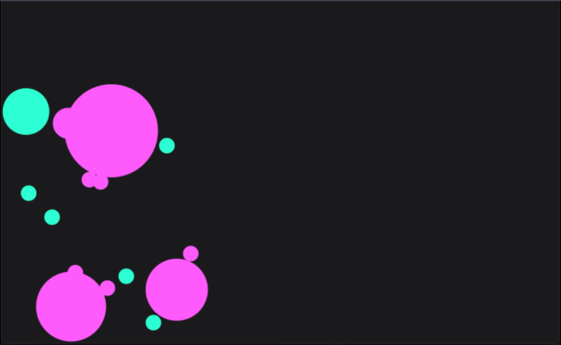## 基础结构

<link rel="stylesheet" href="./style.css" />


<body /> 中，添加 canvas 元素、加载 index.js 文件：

<main>
<canvas id="gameboard"></canvas>
</main>
<script src="./index.js"></script>


style.css 中的代码如下：

* {
box-sizing: border-box;
padding: 0;
margin: 0;
font-family: sans-serif;
}

main {
width: 100vw;
height: 100vh;
background: hsl(0deg, 0%, 10%);
}


hsl(hue, saturation, brightness) 为 css 颜色表示法之一，参数分别为色相，饱和度和亮度，可通过我之前出过的视频进行学习。

## 绘制小球

const canvas = document.getElementById("gameboard");
const ctx = canvas.getContext("2d");

canvas.width = window.innerWidth;
canvas.height = window.innerHeight;

let width = canvas.width;
let height = canvas.height;

ctx.fillStyle = "hsl(170, 100%, 50%)";
ctx.beginPath();
ctx.arc(100, 100, 60, 0, 2 * Math.PI);
ctx.fill();


• 通过 canvas 的 id 获取 canvas 元素对象。
• 通过 canvas 元素对象获取绘图 context， getContext() 需要一个参数，用于表明是绘制 2d 图像，还是使用 webgl 绘制 3d 图象，这里选择 2d。context 就类似是一支画笔，可以改变它的颜色和绘制基本的形状。
• 给 canvas 的宽高设置为浏览器可视区域的宽高，并保存到 widthheight 变量中方便后续使用。
• 给 context 设置颜色，然后调用 beginPath() 开始绘图。
• 使用 arc() 方法绘制圆形，它接收 5 个参数，前两个为圆心的 x、y 坐标，第 3 个为半径长度， 第 4 个和第 5 个分别是起始角度和结束角度，因为 arc() 其实是用来绘制一段圆弧，这里让它画一段 0 到 360 度的圆弧，就形成了一个圆形。这里的角度是使用 radian 形式表示的，0 到 360 度可以用 0 到 2 * Math.PI 来表示。
• 最后使用 ctx.fill() 给圆形填上颜色。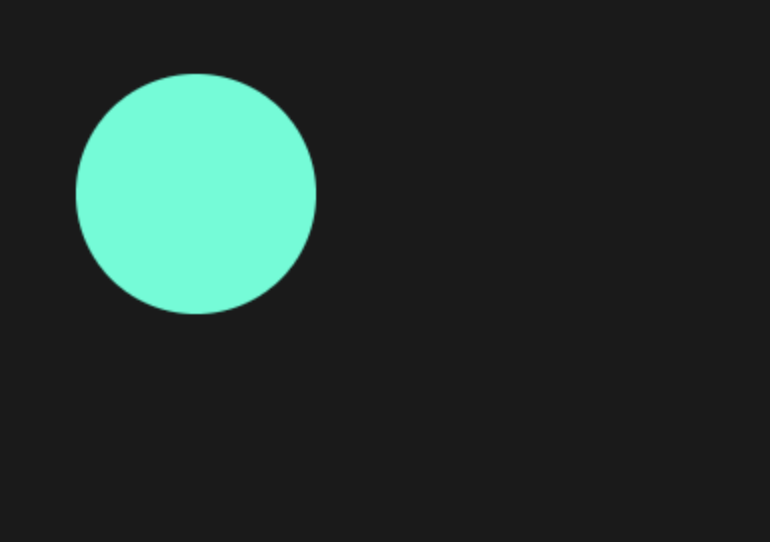## 移动小球

Canvas 进行动画的原理与传统的电影胶片类似，在一段时间内，绘制图像、更新图像位置或形状、清除画布，重新绘制图像，当在 1 秒内连续执行 60 次或以上这样的操作时，即以 60 帧的速度，就可以产生连续的画面。

function process() {
window.requestAnimationFrame(process);
}
window.requestAnimationFrame(process);


let x = 100;
let y = 100;
let vx = 12;
let vy = 25;

function process() {
ctx.fillStyle = "hsl(170, 100%, 50%)";
ctx.beginPath();
ctx.arc(x, y, 60, 0, 2 * Math.PI);
ctx.fill();
window.requestAnimationFrame(process);
}
window.requestAnimationFrame(process);


let startTime;

function process(now) {
if (!startTime) {
startTime = now;
}
let seconds = (now - startTime) / 1000;
startTime = now;

// 更新位置
x += vx * seconds;
y += vy * seconds;

// 清除画布
ctx.clearRect(0, 0, width, height);
// 绘制小球
ctx.fillStyle = "hsl(170, 100%, 50%)";
ctx.beginPath();
ctx.arc(x, y, 60, 0, 2 * Math.PI);
ctx.fill();

window.requestAnimationFrame(process);
}


process() 现在接收当前时间戳作为参数，然后做了下面这些操作：

• 计算上次函数调用与本次函数调用的时间间隔，以秒计，记录本次调用的时间戳用于下一次计算。
• 根据 x、y 方向上的速度，和刚刚计算出来的时间，计算出移动距离。
• 调用 clearRect() 清除矩形区域画布，这里的参数，前两个是左上角坐标，后两个是宽高，把 canvas 的宽高传进去就会把整个画布清除。
• 重新绘制小球。## 重构代码

class Circle {
constructor(context, x, y, r, vx, vy) {
this.context = context;
this.x = x;
this.y = y;
this.r = r;
this.vx = vx;
this.vy = vy;
}

// 绘制小球
draw() {
this.context.fillStyle = "hsl(170, 100%, 50%)";
this.context.beginPath();
this.context.arc(this.x, this.y, this.r, 0, 2 * Math.PI);
this.context.fill();
}

/**
* 更新画布
* @param {number} seconds
*/
update(seconds) {
this.x += this.vx * seconds;
this.y += this.vy * seconds;
}
}


class Gameboard {
constructor() {
this.startTime;
this.init();
}

init() {
this.circles = [
new Circle(ctx, 100, 100, 60, 12, 25),
new Circle(ctx, 180, 180, 30, 70, 45),
];
window.requestAnimationFrame(this.process.bind(this));
}

process(now) {
if (!this.startTime) {
this.startTime = now;
}
let seconds = (now - this.startTime) / 1000;
this.startTime = now;

for (let i = 0; i < this.circles.length; i++) {
this.circles[i].update(seconds);
}
ctx.clearRect(0, 0, width, height);

for (let i = 0; i < this.circles.length; i++) {
this.circles[i].draw(ctx);
}
window.requestAnimationFrame(this.process.bind(this));
}
}

new Gameboard();


• startTime 保存了上次函数执行的时间戳的属性，放到了构造函数中。
• init() 方法创建了一个 circles 数组，里边放了两个示例的小球，这里先不涉及碰撞问题。然后调用 window.requestAnimationFrame() 开启动画。注意这里使用了 bind() 来把 Gameboard 的 this 绑定到回调函数中，以便于访问 Gameboard 中的方法和属性。
• process() 方法也写到了这里边，每次执行时会遍历小球数组，对每个小球进行位置更新，然后清除画布，再重新绘制每个小球。
• 最后初始化 Gameboard 对象就可以开始执行动画了。## 碰撞检测

this.circles = [
new Circle(ctx, 30, 50, 30, -100, 390),
new Circle(ctx, 60, 180, 20, 180, -275),
new Circle(ctx, 120, 100, 60, 120, 262),
new Circle(ctx, 150, 180, 10, -130, 138),
new Circle(ctx, 190, 210, 10, 138, -280),
new Circle(ctx, 220, 240, 10, 142, 350),
new Circle(ctx, 100, 260, 10, 135, -460),
new Circle(ctx, 120, 285, 10, -165, 370),
new Circle(ctx, 140, 290, 10, 125, 230),
new Circle(ctx, 160, 380, 10, -175, -180),
new Circle(ctx, 180, 310, 10, 115, 440),
new Circle(ctx, 100, 310, 10, -195, -325),
new Circle(ctx, 60, 150, 10, -138, 420),
new Circle(ctx, 70, 430, 45, 135, -230),
new Circle(ctx, 250, 290, 40, -140, 335),
];


class Circle {
constructor(context, x, y, r, vx, vy) {
// 其它代码
this.colliding = false;
}
draw() {
this.context.fillStyle = this.colliding
? "hsl(300, 100%, 70%)"
: "hsl(170, 100%, 50%)";
// 其它代码
}
}


( x 1 − x 2 ) 2 + ( y 1 − y 2 ) 2 \sqrt{(x_1 - x_2)^2 + (y_1 - y_2)^2}

x1、y1 和 x2、y2 分别两个小球的圆心坐标。在比较时，可以对半径和进行平方运算，进而省略对距离的开方运算，也就是可以用下方的公式进行比较：

( x 1 − x 2 ) 2 + ( y 1 − y 2 ) 2 ≤ ( r 1 + r 2 ) 2 (x_1 - x_2)^2 + (y_1 - y_2)^2 \leq (r_1 + r_2)^2

r1 和 r2 为两球的半径。

isCircleCollided(other) {
let squareDistance =
(this.x - other.x) * (this.x - other.x) +
(this.y - other.y) * (this.y - other.y);
let squareRadius = (this.r + other.r) * (this.r + other.r);
return squareDistance <= squareRadius;
}


checkCollideWith(other) {
if (this.isCircleCollided(other)) {
this.colliding = true;
other.colliding = true;
}
}


checkCollision() {
// 重置碰撞状态
this.circles.forEach((circle) => (circle.colliding = false));

for (let i = 0; i < this.circles.length; i++) {
for (let j = i + 1; j < this.circles.length; j++) {
this.circles[i].checkCollideWith(this.circles[j]);
}
}
}


for (let i = 0; i < this.circles.length; i++) {
this.circles[i].update(seconds);
}
this.checkCollision();## 边界碰撞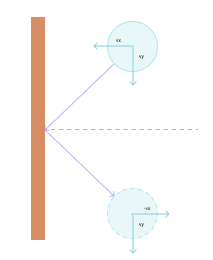checkEdgeCollision() {
this.circles.forEach((circle) => {
// 左右墙壁碰撞
if (circle.x < circle.r) {
circle.vx = -circle.vx;
circle.x = circle.r;
} else if (circle.x > width - circle.r) {
circle.vx = -circle.vx;
circle.x = width - circle.r;
}

// 上下墙壁碰撞
if (circle.y < circle.r) {
circle.vy = -circle.vy;
circle.y = circle.r;
} else if (circle.y > height - circle.r) {
circle.vy = -circle.vy;
circle.y = height - circle.r;
}
});
}


this.checkEdgeCollision();
this.checkCollision();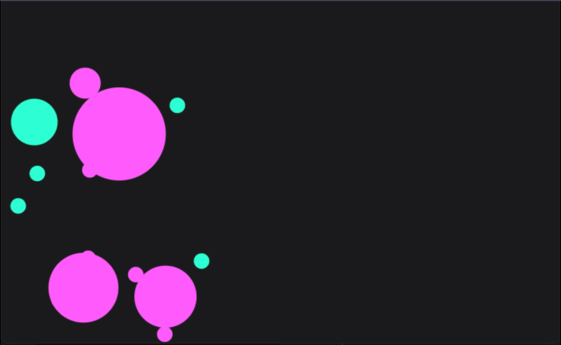## 向量的基本操作

class Vector {
constructor(x, y) {
this.x = x;
this.y = y;
}

/**
* 向量加法
* @param {Vector} v
*/
add(v) {
return new Vector(this.x + v.x, this.y + v.y);
}

/**
* 向量减法
* @param {Vector} v
*/
substract(v) {
return new Vector(this.x - v.x, this.y - v.y);
}

/**
* 向量与标量乘法
* @param {Vector} s
*/
multiply(s) {
return new Vector(this.x * s, this.y * s);
}

/**
* 向量与向量点乘（投影）
* @param {Vector} v
*/
dot(v) {
return this.x * v.x + this.y * v.y;
}

/**
* 向量标准化（除去长度）
* @param {number} distance
*/
normalize() {
let distance = Math.sqrt(this.x * this.x + this.y * this.y);
return new Vector(this.x / distance, this.y / distance);
}
}


## 碰撞处理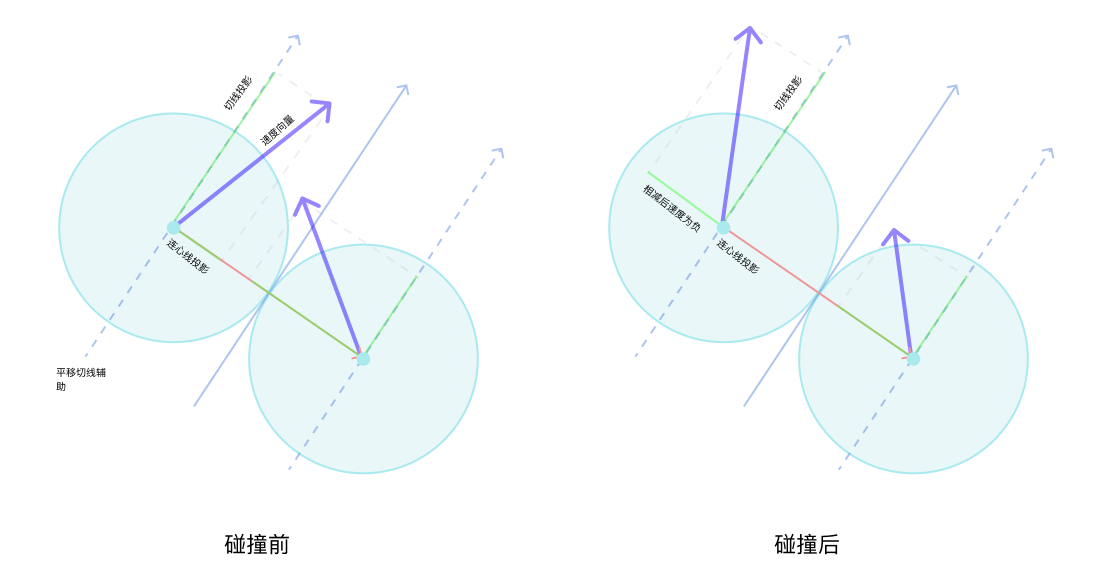##### 动量守恒定律

m 1 v 1 + m 2 v 2 = m 1 v 1 ′ + m 2 v 2 ′ m_1v_1 + m_2v_2 = m_1v_1' + m_2v_2'

##### 动能守恒定律

1 2 m 1 v 1 2 + 1 2 m 2 v 2 2 = 1 2 m 1 v 1 ′ 2 + 1 2 m 2 v 2 ′ 2 \frac{1}{2}m_1v_1^2+\frac{1}{2}m_2v_2^2=\frac{1}{2}m_1v_1'^2+\frac{1}{2}m_2v_2'^2

m1、m2 分别为两小球的质量，v1 和 v2 为两小球碰撞前的速度向量，v1’ 和 v2’ 为碰撞后的速度向量。根据这两个公式可以推导出两小球碰撞后的速度公式：

v 1 ′ = v 1 ( m 1 − m 2 ) + 2 m 2 v 2 m 1 + m 2 v_1'=\frac{v_1(m_1-m_2)+2m_2v_2}{m_1+m_2}

v 2 ′ = v 2 ( m 2 − m 1 ) + 2 m 1 v 1 m 1 + m 2 v_2'=\frac{v_2(m_2-m_1)+2m_1v_1}{m_1+m_2}

v 1 ′ = v 2 v_1'=v_2

v 2 ′ = v 1 v_2'=v_1

class Circle {
constructor(context, x, y, r, vx, vy, mass = 1) {
// 其它代码
this.mass = mass;
}
}


this.circles = [
new Circle(ctx, 30, 50, 30, -100, 390, 30),
new Circle(ctx, 60, 180, 20, 180, -275, 20),
new Circle(ctx, 120, 100, 60, 120, 262, 100),
new Circle(ctx, 150, 180, 10, -130, 138, 10),
new Circle(ctx, 190, 210, 10, 138, -280, 10),
new Circle(ctx, 220, 240, 10, 142, 350, 10),
new Circle(ctx, 100, 260, 10, 135, -460, 10),
new Circle(ctx, 120, 285, 10, -165, 370, 10),
new Circle(ctx, 140, 290, 10, 125, 230, 10),
new Circle(ctx, 160, 380, 10, -175, -180, 10),
new Circle(ctx, 180, 310, 10, 115, 440, 10),
new Circle(ctx, 100, 310, 10, -195, -325, 10),
new Circle(ctx, 60, 150, 10, -138, 420, 10),
new Circle(ctx, 70, 430, 45, 135, -230, 45),
new Circle(ctx, 250, 290, 40, -140, 335, 40),
];


  changeVelocityAndDirection(other) {
// 创建两小球的速度向量
let velocity1 = new Vector(this.vx, this.vy);
let velocity2 = new Vector(other.vx, other.vy);
}


let vNorm = new Vector(this.x - other.x, this.y - other.y);


let unitVNorm = vNorm.normalize();
let unitVTan = new Vector(-unitVNorm.y, unitVNorm.x);


unitVNorm 是连心线方向单位向量，unitVTan 是切线方向单位向量，切线方向其实就是把连心线向量的 x、y 坐标互换，并把 y 坐标取反。根据这两个单位向量，使用点乘计算小球速度在这两个方向上的投影：

let v1n = velocity1.dot(unitVNorm);
let v1t = velocity1.dot(unitVTan);

let v2n = velocity2.dot(unitVNorm);
let v2t = velocity2.dot(unitVTan);


let v1nAfter = (v1n * (this.mass - other.mass) + 2 * other.mass * v2n) / (this.mass + other.mass);
let v2nAfter = (v2n * (other.mass - this.mass) + 2 * this.mass * v1n) / (this.mass + other.mass);


v1nAfterv2nAfter 分别是两小球碰撞后的速度，现在可以先判断一下，如果 v1nAfter 小于 v2nAfter，那么第 1 个小球和第 2 个小球会越来越远，此时不用处理碰撞：

if (v1nAfter < v2nAfter) {
return;
}


let v1VectorNorm = unitVNorm.multiply(v1nAfter);
let v1VectorTan = unitVTan.multiply(v1t);

let v2VectorNorm = unitVNorm.multiply(v2nAfter);
let v2VectorTan = unitVTan.multiply(v2t);


let velocity1After = v1VectorNorm.add(v1VectorTan);
let velocity2After = v2VectorNorm.add(v2VectorTan);


this.vx = velocity1After.x;
this.vy = velocity1After.y;

other.vx = velocity2After.x;
other.vy = velocity2After.y;


checkCollideWith(other) {
if (this.isCircleCollided(other)) {
this.colliding = true;
other.colliding = true;
this.changeVelocityAndDirection(other); // 在这里调用
}
}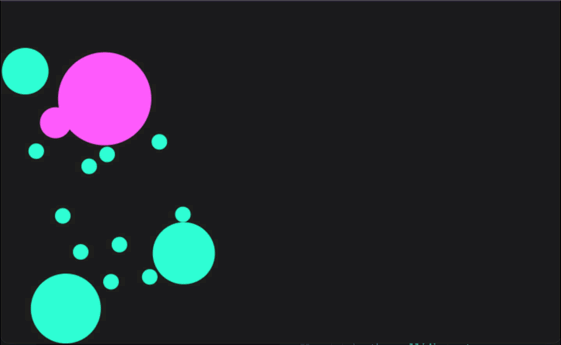## 非弹性碰撞

  checkEdgeCollision() {
const cor = 0.8;                  // 设置恢复系统
this.circles.forEach((circle) => {
// 左右墙壁碰撞
if (circle.x < circle.r) {
circle.vx = -circle.vx * cor; // 加上恢复系数
circle.x = circle.r;
} else if (circle.x > width - circle.r) {
circle.vx = -circle.vx * cor; // 加上恢复系数
circle.x = width - circle.r;
}

// 上下墙壁碰撞
if (circle.y < circle.r) {
circle.vy = -circle.vy * cor; // 加上恢复系数
circle.y = circle.r;
} else if (circle.y > height - circle.r) {
circle.vy = -circle.vy * cor; // 加上恢复系数
circle.y = height - circle.r;
}
});
}


class Circle {
constructor(context, x, y, r, vx, vy, mass = 1, cor = 1) {
// 其它代码
this.cor = cor;
}
}

class Gameboard {
init() {
this.circles = [
new Circle(ctx, 30, 50, 30, -100, 390, 30, 0.7),
new Circle(ctx, 60, 180, 20, 180, -275, 20, 0.7),
new Circle(ctx, 120, 100, 60, 120, 262, 100, 0.3),
new Circle(ctx, 150, 180, 10, -130, 138, 10, 0.7),
new Circle(ctx, 190, 210, 10, 138, -280, 10, 0.7),
new Circle(ctx, 220, 240, 10, 142, 350, 10, 0.7),
new Circle(ctx, 100, 260, 10, 135, -460, 10, 0.7),
new Circle(ctx, 120, 285, 10, -165, 370, 10, 0.7),
new Circle(ctx, 140, 290, 10, 125, 230, 10, 0.7),
new Circle(ctx, 160, 380, 10, -175, -180, 10, 0.7),
new Circle(ctx, 180, 310, 10, 115, 440, 10, 0.7),
new Circle(ctx, 100, 310, 10, -195, -325, 10, 0.7),
new Circle(ctx, 60, 150, 10, -138, 420, 10, 0.7),
new Circle(ctx, 70, 430, 45, 135, -230, 45, 0.7),
new Circle(ctx, 250, 290, 40, -140, 335, 40, 0.7),
];
}
}


v 1 ′ = m 1 v 1 + m 2 v 2 + m 2 C R ( v 2 − v 1 ) m 1 + m 2 v_1'=\frac{m_1v_1+m_2v_2+m2C_R(v_2-v_1)}{m_1+m_2}

v 2 ′ = m 1 v 1 + m 2 v 2 + m 1 C R ( v 1 − v 2 ) m 1 + m 2 v_2'=\frac{m_1v_1+m_2v_2+m1C_R(v_1-v_2)}{m_1+m2}

let cor = Math.min(this.cor, other.cor);
let v1nAfter =
(this.mass * v1n + other.mass * v2n + cor * other.mass * (v2n - v1n)) /
(this.mass + other.mass);

let v2nAfter =
(this.mass * v1n + other.mass * v2n + cor * this.mass * (v1n - v2n)) /
(this.mass + other.mass);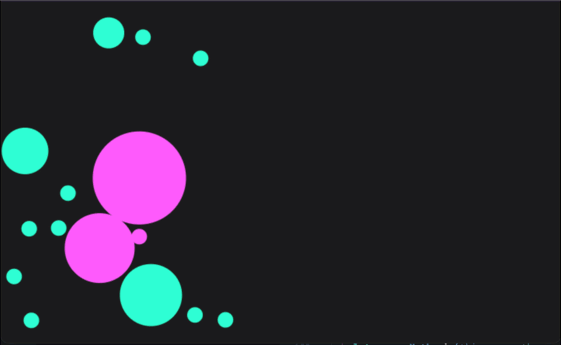## 重力

const gravity = 980;

class Circle {
update(seconds) {
this.vy += gravity * seconds; // 重力加速度
this.x += this.vx * seconds;
this.y += this.vy * seconds;
}
}## 总结

• 使用 context 绘制小球。
• 搭建 Canvas 动画基础结构，主要使用 window.requestAnimationFrame方法反复执行回调函数。
• 移动小球，通过小球的速度和函数执行时的时间戳来计算移动距离。
• 碰撞检测，通过比对两个小球的距离和它们半径的和。
• 边界碰撞的检测和方向改变。
• 小球之间的碰撞，应用速度公式和向量操作计算出碰撞后的速度和方向。
• 利用恢复系数实现非弹性碰撞。
• 添加重力效果。

https://github.com/zxuqian/html-css-examples/tree/master/35-collision-physics

01-21798002-011万+
05-27448
01-311279
08-1175万+
02-02271
11-013067
06-042万+
02-16294
11-14795
12-155129
06-109178
12-2837
12-09453
06-242444
12-011万+
02-04197
©️2020 CSDN 皮肤主题: 技术黑板 设计师:CSDN官方博客